Courses

# Magnetic Field due to Electric Current Class 10 Notes | EduRev

## Class 10 : Magnetic Field due to Electric Current Class 10 Notes | EduRev

The document Magnetic Field due to Electric Current Class 10 Notes | EduRev is a part of the Class 10 Course Science Class 10.
All you need of Class 10 at this link: Class 10

Magnetic Effects Of Current

Magnetism

• The property, due to which a substance attracts pieces of iron, nickel and cobalt towards itself, is called magnetism.
• A naturally occurring iron ore (black iron oxide - Fe2O3) having properties of attracting iron pieces, was found in Magnesia in the upper part of Greece. The name magnetism has been taken from the name of that place.
• The substance having the property of magnetism is called a magnetic substance, and the body made up of a magnetic substance, is called a magnet.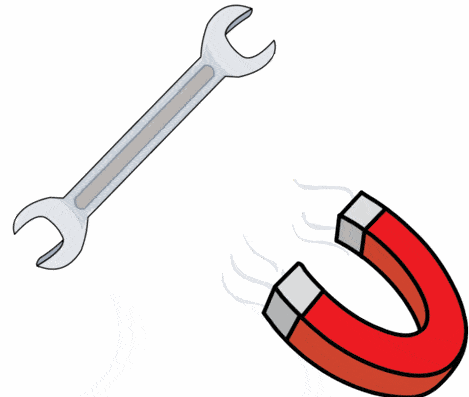Magnet
• Magnets are found in various shapes and sizes. A bar magnet is a long rectangular bar of uniform cross-section, which can attract pieces of iron, steel, cobalt and nickel. The magnet can be natural or artificial.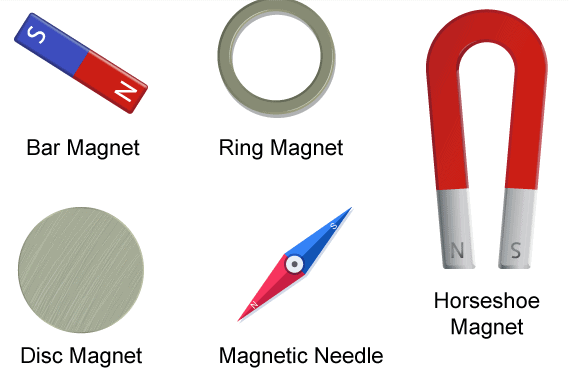Different Types of Magnet

Poles of a Magnet

• When a magnet is dipped in iron filings, then maximum filings stick to its ends, and almost no filings stick to its centre. It means that in magnets, centres of attraction are located near the ends only.
• These centres of attraction near the ends of a magnet are called poles. Since a magnet has two poles, hence it is also called a magnetic dipole.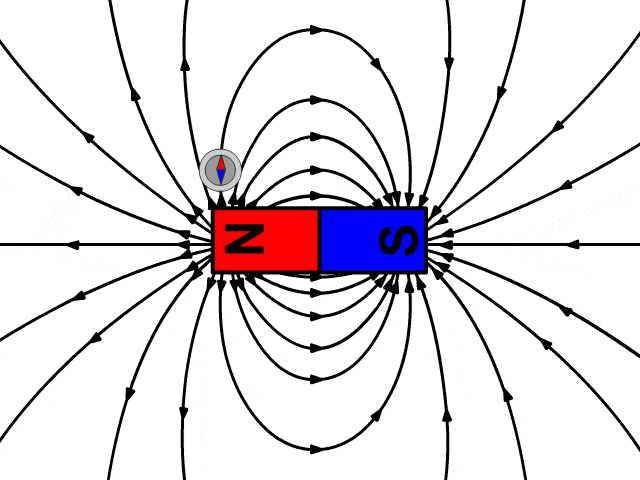Magnetic Dipole with Field Lines
• When this magnet is freely suspended, its two ends point in the north-south direction.
• The pole near the end pointing towards North (north-seeking end) is called North pole
• The pole near the end pointing towards South (south-seeking end) is called South pole.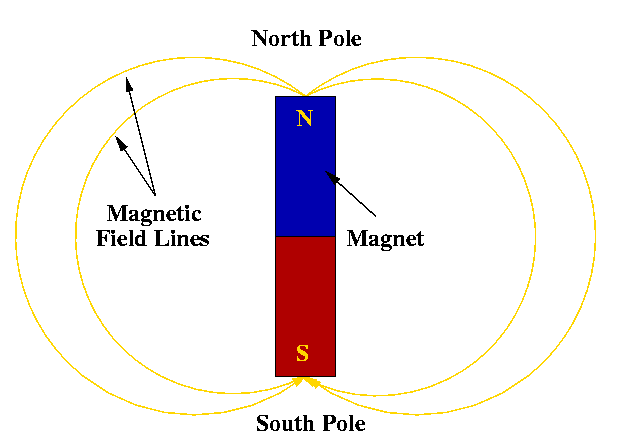• The magnetic poles exert forces on each other. Like poles repel each other, i.e., a north pole will repel another north pole or a south pole will repel another south pole. Unlike poles attract each other, i.e., a south pole will attract a north pole and vice versa.

Magnetic Field of Earth

• Earth behaves as a huge magnet (or a giant solenoid). The source of this huge magnetism is given as the molten charged metallic fluid giving rise to a current flowing inside the core of the earth. This core has a radius of about 3500 km (Earth's radius is 6400 km).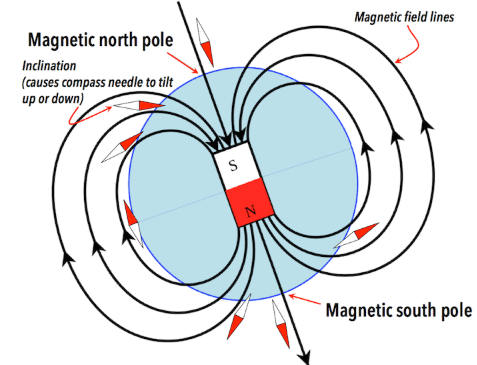Earth's Magnetic Field

• Its strength is of the order of one gauss. The shape of the earth's magnetic field resembles with that of a bar magnet of length one-fifth of earth's diameter buried at its centre.
• It is believed that earth's magnetism is due to the magnetic effect of current, which is flowing in the molten core at the centre of the earth. Hence, the earth is a huge electromagnet.

Magnetic Field and Magnetic Field Lines (Magnetic Lines of force)

Magnetic Field

It is the space around a magnetic pole or a magnet in which another magnetic pole or magnet experiences its effect. The magnetic field is a quantity which has both direction and magnitude.

Magnetic Field Lines (Magnetic Lines of Force)

• A magnetic line of force is a line, straight or curved, in the magnetic field tangent to which at any point gives the direction of the magnetic field at that point.
Or
A line such that the tangent at any point on it gives the direction of the magnetic field at that point is called a magnetic field or magnetic line of force.
• A free unit north pole (test pole) will move along the magnetic line of force in the direction of the field if it is free to do so.
• The direction of the magnetic line of force at any point in the direction of the force acting on the unit (north) pole (unit magnetic pole) when placed at that point.
• Since a free unit north pole (test pole) will move away from a north (N) pole, magnetic lines of force have outward direction [Fig. (a)].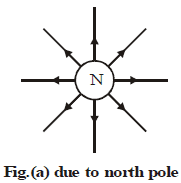• Since the free unit north pole will move towards a south (S) pole, magnetic lines of force have inward direction [Fig. (b)].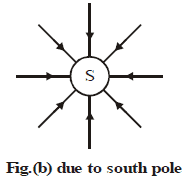• When moved along the line of force, a small magnetic compass always sets itself parallel to the line of force.

Magnetic lines of force have the following properties:

• All field lines are closed curves. They come out of magnet from the side of the north pole and go into it on the side of the south pole. i.e. They start from a north (positive) pole and end at a south (negative) pole. They continue inside the magnet too. Inside the magnet, the direction of field lines is from its south pole to its north pole.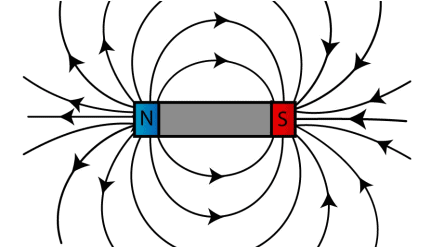Magnetic Field Lines Around a Bar Magnet
• They are always normal to the surface of the magnet at every point.
• Two lines of force do not intersect each other. If they intersect at a point, it would mean that compass needle placed at the point of intersection would point towards two directions at that point which is not possible.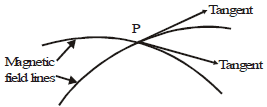• The field lines are close together near the poles and spread out away from them. The field is stronger where the field lines are more closely spaced. So the field is stronger near the poles than at other points.
• The number of magnetic lines of force passing normally per unit area about a point gives the intensity of the magnetic field at that point.

Try yourself:Inside the magnet, the field lines moves

Magnetic Field Due to a Current Carrying Conductor

• Oersted (1820) was the first to discover the magnetic effect of current.
• He found that if a compass needle is placed near a current-carrying wire, a needle gets deflected. He said that a compass needle is a tiny magnet and can be deflected only by some other magnetic field, hence a current-carrying wire produces some magnetic field around itself.
• Magnetic effect of current is also known as electromagnetism. Magnetic effect of current is very useful in electric motors, generators, telephone etc.
• Experimental arrangement used by Oersted is shown in Fig: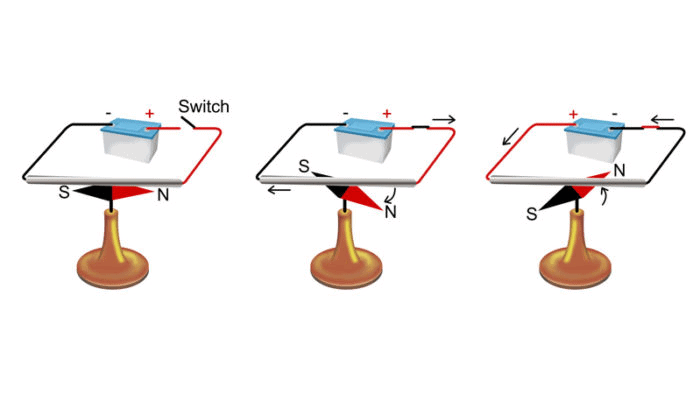• A straight wire is connected to a battery Ba and switch. The wire is held horizontally north-south over a magnetic needle.
• In this arrangement, when the key is closed, current flows in the wire in the direction, as shown in fig. The north pole of the needle gets deflected towards west. When the key is taken out, and current in the wire becomes zero, the needle returns to its initial position (S - N). This shows that a magnetic field is associated with an electric current.
• When the direction of current in the wire is reversed, the direction of deflection of the needle also gets reversed. If the direction of current is kept the same and the wire is put under the needle then, the direction of deflection of the needle again gets reversed.
• Amount of deflection depends on the distance of the needle from the current-carrying wire.
• But we know that a magnetic needle is deflected by a magnetic field only. Hence we can conclude that current flowing in a wire gives rise to some magnetic field around it.
• Position of the wire (conductor) carrying the current, direction of current and direction of deflection of the needle can be related by SNOW rule given below.

Note: If current flows in the conductor from South towards North, with conductor, kept over the needle, then North pole of the needle will be deflected towards West.

Magnetic Field Due to Current through a Straight Conductor (Wire) and a Circular Coil
When the current-carrying conductor is Straight, Magnetic Field is Circular

• It means that when the current flows in a straight wire, the magnetic field produced has circular lines of force surrounding the wire, having their centres at the wire, as shown in Fig. This can be shown by sprinkling iron filings on the cardboard C.
• When current flows through the conductor, iron filings get magnetised and now if the cardboard C is tapped gently iron filings arrange themselves in the circles around the wire.
• Hence we can say that magnetic lines of force around a straight current-carrying conductor are circular. The plane of circular lines is perpendicular to the length of the wire. Arrows mark Their direction.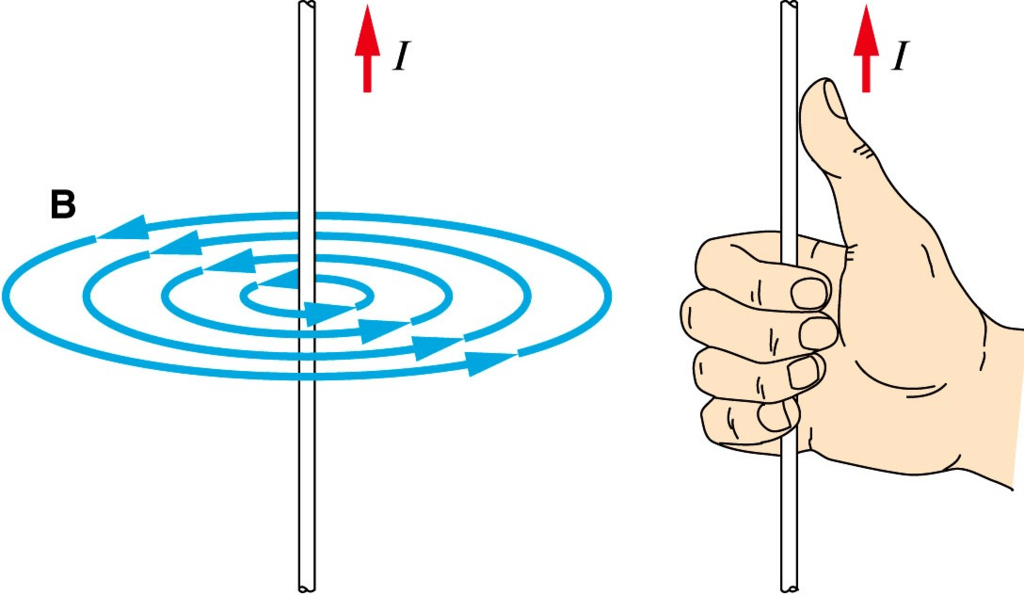Current Straight, Magnetic Field is Circular
• When current I flows through a straight wire, the magnetic field strength (B) at a small distance r from it is given by: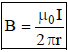• From the above expression, we see that magnitude of the magnetic field produced by a straight current-carrying wire at a given point is:
(i) Directly proportional to the quantity of current flowing through the wire.
(ii) Inversely proportional to the distance of that point from the wire. Thus, if the current is more, the magnetic field will be stronger and vice versa.
• The direction of Magnetic Field
The direction of the magnetic lines of force is related to the direction of the current by the right-hand thumb rule.
• This rule states that:
Curl the four fingers of the right hand on the palm, keeping the thumb stretched out at right angles. The thumb is straight, and the fingers are circular, then
(i) If thumb represents the direction of the current in the straight wire, then curling of fingers represents the direction of the circular magnetic lines of force. (Fig. (a))
(ii) If curled fingers represent the direction of the current in the circular wire, then thumb represents the direction of the straight magnetic lines of force. (Fig. (b))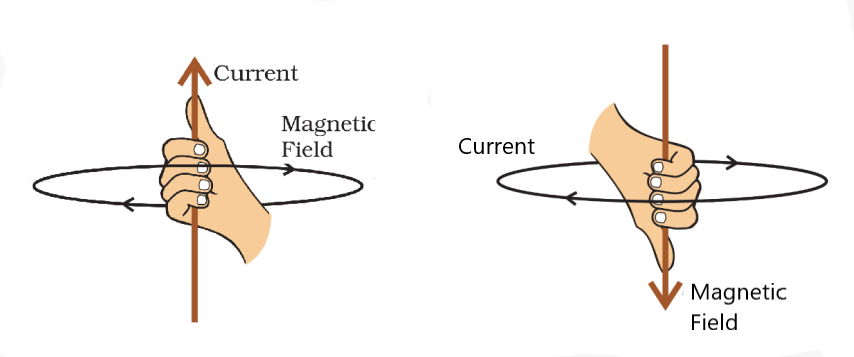(a) and (b) Right Hand Thumb Rule for Direction of Magnetic Field
• Maxwell's right-hand thumb rule is also known as Maxwell corkscrew rule. Corkscrew is a device consisting of a handle and a spiral metal rod, as shown in fig.(c).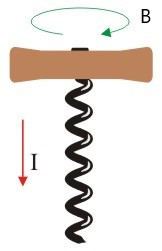(c) Cork Screw
• When the corkscrew is moved in the direction of the current, then the direction in which its handle is turned gives us the magnetic field direction. In short, we can say that when current flows vertically upward, then the direction of the magnetic field produced is anticlockwise and when current flows vertically downward, then the direction of the magnetic field is clockwise.

Try yourself:The pattern of the magnetic field produced by the straight current carrying conducting wire is

Magnetic Field Due to Current through a Circular Coil

When Current is Circular, Magnetic Field is Straight

• When the current flows in a circular wire (coil), the magnetic field produced has straight lines of force near the centre of the coil, as shown in Fig. The parallel lines are in a plane perpendicular to the plane of the coil. The arrows mark their direction.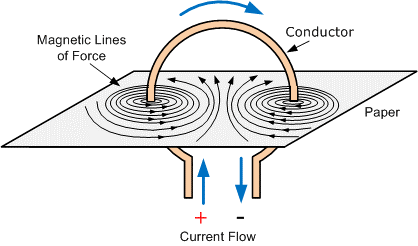Current Circular, Magnetic Field is Straight
• Straight lines at the middle of the coil are due to the fact that the magnetic lines of force surround each small segment of the circular coil. At the centre of the coil, all lines of force add to each other resulting in the increase in strength.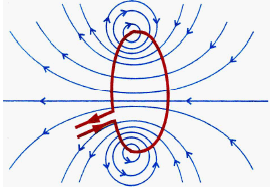Magnetic Lines of Force around a Circular Coil
• It is found that magnitude of the magnetic field (B) at the centre of a circular coil carrying currents is directly proportional to the amount of current flowing through the wire (I), inversely proportional to the radius of the coil (r) and directly proportional to the number of turns in the coil.
Mathematically: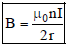• Here, n is the number of turns of the coil.

Magnetic Field Due to Current in a Solenoid

• A coil of many turns of wire wrapped in the shape of a cylinder is called a solenoid, i.e., a solenoid is a long cylindrical coil wound over a hollow cylinder (non conducting). It is shown in Fig.(a).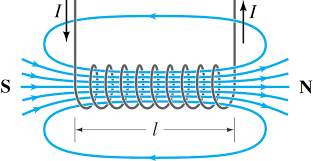(a) Solenoid carrying Current and Polarity of its Faces
• A solenoid differs from a circular coil in that the length of the solenoid is much greater than its diameter.
• A solenoid behaves as if a large number of coils have been put one behind the other on the same axis over a length, i.e., the magnetic field produced by a current-carrying solenoid is similar to the magnetic field produced by a bar magnet. Current in solenoid produces a magnetic field in each turn, which becomes additive.
• The polarity of the magnetic field exists only at the ends of the solenoid, as shown in Fig.(a).
• One end of the coil acts as a north pole while the other end acts as a south pole.
• Inside the solenoid, the magnetic field is uniform (same at all points). It is represented by parallel and straight field lines. Magnetic field outside the solenoid is non-uniform.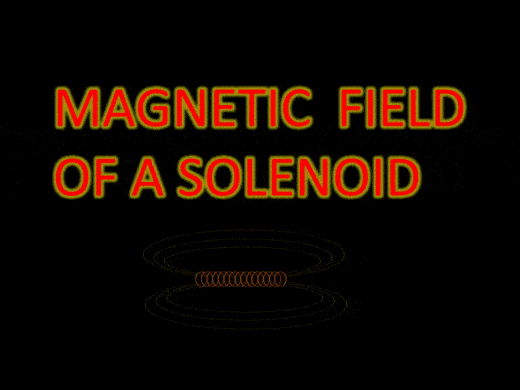Magnetic Field Lines around a Solenoid
• Magnetic lines of force inside the solenoid are from south pole to north pole, while outside the solenoid these lines are from north pole to south pole.
• The rule for Polarity at the ends: If at any end, the current in the coil (or loop) is clockwise, the face of the coil towards the observer behaves as a south pole. [Fig. (b)]
• If the current in the coil is anticlockwise at any end, the face of the coil towards the observer behaves as a north pole. [Fig. (c)].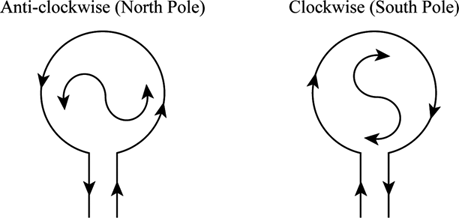(b) & (c) Direction of Current in Loop
• Fig. (d) shows the magnetic field around a current-carrying solenoid.
Magnetic field intensity B depends upon the following factors:
(i) Number of turns per unit length (n) of the solenoid and B ∝ n
(ii) Strength of the current (I) in the solenoid and B ∝ I
(iii) Nature (relative permeability, μ) of the core material of the solenoid.
B ∝ μr or B ∝ μr nI or
B = μ0μrnI
Where μ0 represents permeability of free space.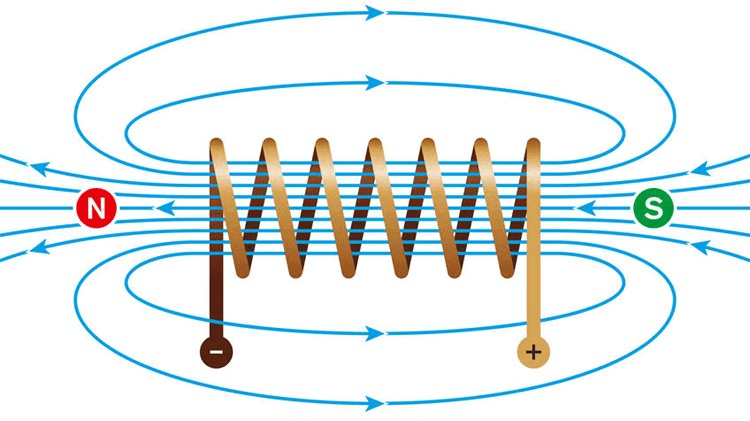(d) Magnetic Field around a Solenoid: The Field is Straight inside it.

Note: Permeability is a magnetic property of material related to magnetism.

Ques: How are the fields of a current-carrying solenoid and coil different?
Sol: The field inside a current-carrying solenoid is quite uniform for the most part. It only decreases near the ends. The field of a current-carrying coil is not uniform. It changes with distance from the centre.

Try yourself:The factors on which one magnetic field strength produced by current carrying solenoids depends are

Electromagnet

• The strong magnetic field that can be produced due to the current flow can be used to magnetise a piece of a magnetic material like a steel rod, soft iron etc. when placed inside the coil. Magnet so produced is called an electromagnet.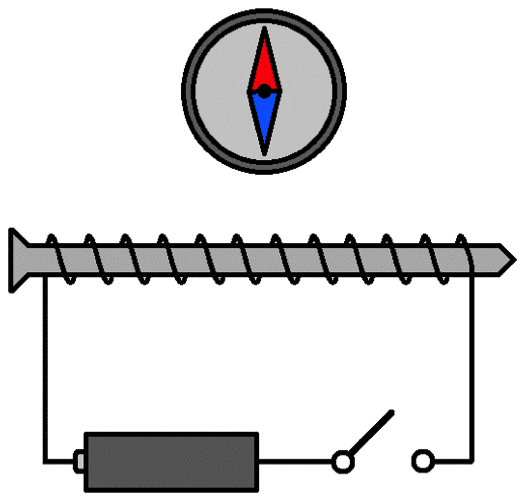Electromagnet
• Thus, an electromagnet consists of many turns of insulated copper wire wound over a soft iron core or horse-shoe-magnet.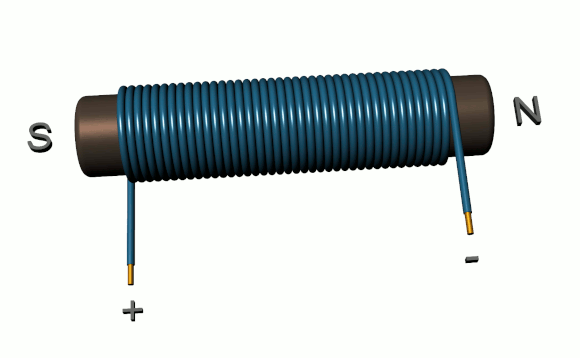Electromagnet

Strength of the electromagnet depends on:
(i) Number of turns in the coil: If the number of turns in the coil is more, the electromagnet will be strong.
(ii) Current flowing in the coil: Strength of electromagnet is directly proportional to the current flowing through the coil.
(iii) Length of the air gap between the poles: Strength of the electromagnet is inversely proportional to the distance between its poles.

• Electromagnets are used to extract bullets from the body, to lift big machines and in electronic devices.
• Large values of mr n and I can produce a very strong magnetic field inside the solenoid.
• Electromagnets are better than permanent magnets as their strength can be controlled by changing the number of turns in the coil or by changing the current flowing through the coil.
• Permanent magnets are usually made of some alloys of steel because permanent magnets made from alloys are very strong than those made from ordinary steel.

Table: Difference between an Electromagnet and a Permanent Magnet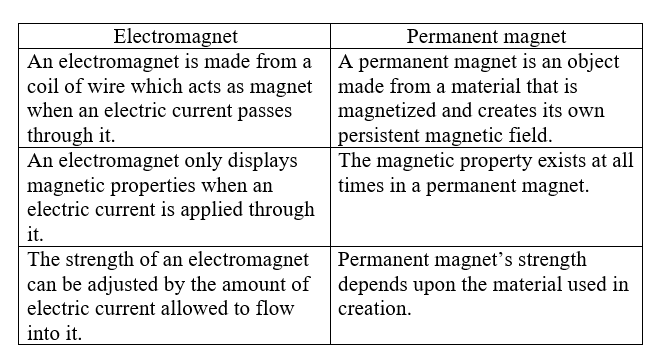Do You Know

• Hard steel, alnico (an alloy of aluminium, nickel, cobalt, iron and nipermag (an alloy of aluminium, iron and titanium) are used to make permanent magnets.
• Soft iron is used to make electromagnets.
• A steel rod can be made a permanent magnet if it is placed inside a solenoid carrying direct current.
• Permanent magnets are used in loudspeakers, galvanometers, voltmeters, ammeters and speedometers.
• Permanent magnet can be damagnetised by heating it.
Offer running on EduRev: Apply code STAYHOME200 to get INR 200 off on our premium plan EduRev Infinity!

## Science Class 10

85 videos|255 docs|129 tests

,

,

,

,

,

,

,

,

,

,

,

,

,

,

,

,

,

,

,

,

,

;